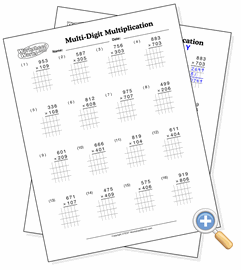# Multi-Digit Multiplication

## Handling ZerosPractice multiple-digit multiplication when zero digits are present

A sometimes challenging situation in multi-digit multiplication is when the second parameter contains a zero. If following the rules strictly, students will fill in that row with zeros. But the simplest way to handle it is to skip a row and bump the next result over two digits instead of one. All of these problems have a zero somewhere in the second parameter to provide practice in handling this scenario.